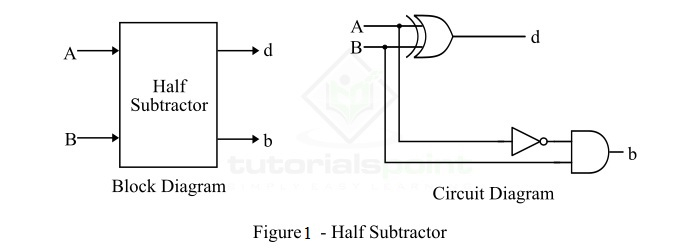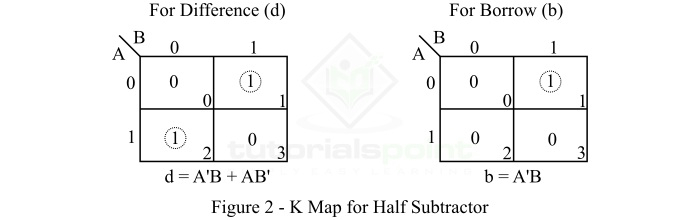# Half Subtractor in Digital Electronics

In digital electronics, a subtractor is a combinational logic circuit that can perform the subtraction of two number (binary numbers) and produce the difference between them. It is a combinational circuit that means its output depends on its present inputs only. Although, in practice, the subtraction of two binary number is accomplished by taking the 1's or 2's compliment of the subtrahend and adding it to the minuend. In this way, the subtraction operation of binary numbers can be converted into simple addition operation which makes hardware construction simple and less expensive. There are two types of subtractors namely, Half Subtractor and Full Subtractor.

In this article, we will discuss the half subtractor, its basic definition, circuit diagram, truth table, characteristic equation, etc. So let's begin with the basic definition of half subtractor.

## What is a Half-Subtractor?

A half-subtractor is a combinational logic circuit that have two inputs and two outputs (i.e. difference and borrow). The half subtractor produces the difference between the two binary bits at the input and also produces a borrow output (if any). In the subtraction (A-B), A is called as Minuend bit and B is called as Subtrahend bit. The block diagram and logic circuit diagram of the half subtractor is shown in Figure-1.Hence, from the logic circuit diagram, it is clear that a half subtractor can be realized using an XOR gate together with a NOT gate and an AND gate.

In the half subtractor as shown in figure-1, A and B are the inputs, d and b are the outputs. Where, d indicates the difference and b indicates the borrow output. The borrow output (b) is the signal that tells the next stage that a 1 has been borrowed.

## Operation of Half Subtractor

Now, let us understand the operation of the half subtractor circuit. Half subtractor performs its operation to find the difference of two binary digits according to the rules of binary subtraction, which are as follows −

The output borrow of b is zero (0) as long as the minuend bit (A) is greater than or equal to the subtrahend bit (B), i.e. A ≥ B. The output borrow is a 1 when A = 0 and B = 1.

From the logic circuit diagram of the half subtractor, it is clear that the difference bit (d) is obtained by the XOR operation of the two inputs A and B, and the borrow bit is obtained by AND operation of the compliment of the minuend (A') with the subtrahend (B).

## Truth Table of Half Subtractor

The following is the truth table the half-subtractor −

Inputs Outputs
A B d (Difference) b (Borrow)
0 0 0 0
0 1 1 1
1 0 1 0
1 1 0 0

## K-Map for Half Subtractor

We can use the K-Map (or Karnaugh Map), a method for simplifying Boolean algebra, to determine equations of the difference bit (d) and the output borrow (b).

The K-Map simplification for half subtractor is shown in Figure-2.## Characteristic Equation of Half Subtractor

The characteristic equations of the half subtractor, i.e. equations of the difference bit (d) and the output borrow bit (b) are obtained by following the rules of binary subtraction. These equations are given as follows −

The difference bit (d) of the half subtractor is given by XORing the two inputs A and B. Therefore,

$$\mathrm{Difference,\, d=A\oplus B=A'B+AB'}$$

The borrow (b) of the half subtractor is the AND of A’ (compliment of A) and B. Therefore,

$$\mathrm{Borrow,\, b=A'B}$$

## Applications of half Subtractor

The following are some important applications of half subtractor −

• Half subtractor is used in ALU (Arithmetic Logic Unit) of processors.

• Half subtractor can also be used in amplifiers to compensate the sound distortion.

• It is also used to decrease the force of radio signals or audio signals.

• Half subtractor is also used to increase or decrease operators.

## Conclusion

From the above discussion, we can conclude that a half subtractor is a combinational logic circuit that can calculate the difference of two binary digits. A half subtractor can only be used to subtract the LSB (Least Significant Bit) of the subtrahend from the LSB of the minuend when one binary number is subtracted from another binary number.